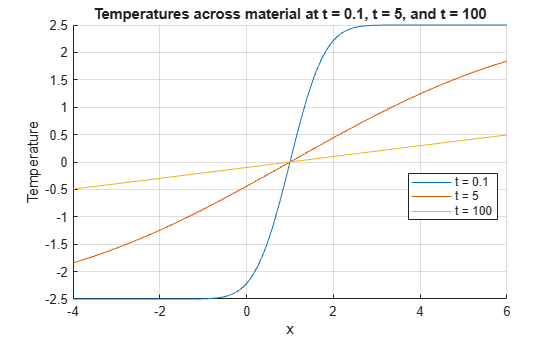# erf

Error function

## Syntax

erf(x)

## Description

example

erf(x) returns the Error Function evaluated for each element of x.

## Examples

collapse all

Find the error function of a value.

erf(0.76)
ans = 0.7175 

Find the error function of the elements of a vector.

V = [-0.5 0 1 0.72]; erf(V)
ans = 1×4 -0.5205 0 0.8427 0.6914 

Find the error function of the elements of a matrix.

M = [0.29 -0.11; 3.1 -2.9]; erf(M)
ans = 2×2 0.3183 -0.1236 1.0000 -1.0000 

The cumulative distribution function (CDF) of the normal, or Gaussian, distribution with standard deviation $\sigma$ and mean $\mu$ is

$\varphi \left(x\right)=\frac{1}{2}\left(1+erf\left(\frac{x-\mu }{\sigma \sqrt{2}}\right)\right).$

Note that for increased computational accuracy, you can rewrite the formula in terms of erfc . For details, see Tips.

Plot the CDF of the normal distribution with $\mu =0$ and $\sigma =1$.

x = -3:0.1:3; y = (1/2)*(1+erf(x/sqrt(2))); plot(x,y) grid on title('CDF of normal distribution with \mu = 0 and \sigma = 1') xlabel('x') ylabel('CDF')Where $u\left(x,t\right)$ represents the temperature at position $x$ and time $t$, the heat equation is

$\frac{\partial u}{\partial t}=c\frac{{\partial }^{2}u}{\partial {x}^{2}},$

where $c$ is a constant.

For a material with heat coefficient $k$, and for the initial condition $u\left(x,0\right)=a$ for $x>b$ and $u\left(x,0\right)=0$ elsewhere, the solution to the heat equation is

$u\left(x,t\right)=\frac{a}{2}\left(erf\left(\frac{x-b}{\sqrt{4kt}}\right)\right).$

For k = 2, a = 5, and b = 1, plot the solution of the heat equation at times t = 0.1, 5, and 100.

x = -4:0.01:6; t = [0.1 5 100]; a = 5; k = 2; b = 1; figure(1) hold on for i = 1:3 u(i,:) = (a/2)*(erf((x-b)/sqrt(4*k*t(i)))); plot(x,u(i,:)) end grid on xlabel('x') ylabel('Temperature') legend('t = 0.1','t = 5','t = 100','Location','best') title('Temperatures across material at t = 0.1, t = 5, and t = 100')## Input Arguments

collapse all

Input, specified as a real number, or a vector, matrix, or multidimensional array of real numbers. x cannot be sparse.

Data Types: single | double

collapse all

### Error Function

The error function erf of x is

$\text{erf(}x\text{)=}\frac{2}{\sqrt{\pi }}{\int }_{0}^{x}{e}^{-{t}^{2}}dt.$

## Tips

• You can also find the standard normal probability distribution using the function normcdf (Statistics and Machine Learning Toolbox). The relationship between the error function erf and normcdf is

$\text{normcdf}\left(x\right)=\frac{1}{2}\left(1-\text{erf}\left(\frac{-x}{\sqrt{2}}\right)\right).$

• For expressions of the form 1 - erf(x), use the complementary error function erfc instead. This substitution maintains accuracy. When erf(x) is close to 1, then 1 - erf(x) is a small number and might be rounded down to 0. Instead, replace 1 - erf(x) with erfc(x).

## Version History

Introduced before R2006a